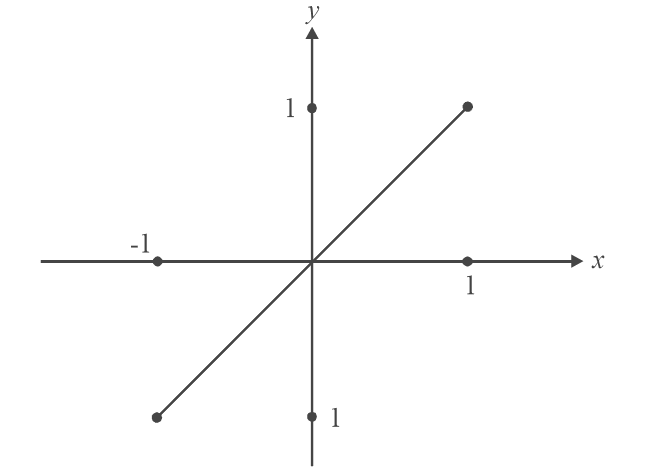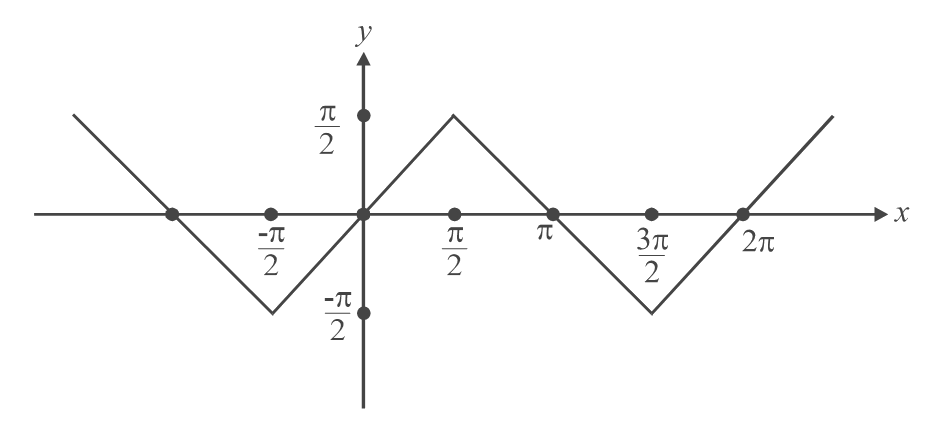# Functions Set 3

Go back to  'SOLVED EXAMPLES'

Example – 4

For real x, find the condition on a, b, c such that the function

f(x) = \begin{align}\frac{{(x - a)(x - b)}}{{(x - c)}}\,\,\,\,\,\,\,\,\,\,\,\,(\mathbb{R} - \{ c\} \to \mathbb{R})\end{align}  is onto

Solution:    Let y = f(x) =\begin{align} \frac{{(x - a)(x - b)}}{{(x - c)}}\end{align}  . Since the co-domain for this function has been specified has $$\mathbb{R}$$, we require the range to be $$\mathbb{R}$$ also if f(x) is to be onto. Hence we require y to take on all real values.

Now   $$y(x - c) = (x - a)(x - b)$$

$\Rightarrow {x^2} - (a + b + y)x + (ab + cy) = 0$

We again follow the method discussed earlier. For x to be real, the discriminant should be non-negative.

$\Rightarrow {(a + b + y)^2} - 4(ab + cy) \ge 0\qquad\qquad\qquad ....(*)$

For the function f to be onto, we require that each y have a real pre-image x. This is only possible if that y satisfies the constraint (*).  Hence, this constraint, or this inequality, should be true for all real y. Rearanging as a quadratic in y

$\Rightarrow {y^2} + 2(a + b - 2c)y + {(a - b)^2} \ge 0\qquad\qquad\qquad ...(**)$

As we saw in Q3, for the LHS of (**) to be always non-negative, we require its graph to lie above the x-axis (or touching it, at the most). If it goes below the axis, the LHS will become negative.

Hence we require the discriminant for (**) to be non-positive. i.e. D < 0

$\Rightarrow {\left( {2(a + b - 2c)} \right)^2} - 4{(a - b)^2}\le 0$

$\Rightarrow {(a + b)^2} + 4{c^2} - 4(a + b)c - {(a - b)^2} \le 0$

$\Rightarrow ab + {c^2} - (a + b)c \le 0$

As in Q3., we can treat the LHS above as a quadratic in ‘c’. LHS < 0 implies that ‘c’ must lie within the roots of this quadratic expression, which are

$\frac{{(a + b) \pm \sqrt {{{(a + b)}^2} - 4ab} }}{2} = \frac{{(a + b) \pm (a - b)}}{2} = a,b$

There is no loss of generality in assuming that a > b since the expression for f(x) is symmetric about ‘a’ and ‘b’.

Hence, we get the constraint on a, b, c as

a < c < b

Question: Why cannot c be equal to a or b?

Example – 5

Plot the graphs for the following:

(a) $$y = \sin \left( {{{\sin }^{ - 1}}x} \right)$$     (b)  $$y = {\sin ^{ - 1}}(\sin x)$$

Solution: By observation, you will have a tendency to say that since y is a composition of two inverse functions, which should cancel out, the output should be y = x, which is a straight line. But we have to be more cautious:

(a)    The inner function, $${\sin ^{ - 1}}x$$, is defined only for x $$\in$$ [–1, 1]. For these values of x, $$\sin ({\sin ^{ - 1}}x)$$  will give back x again. Hence, the graph is the identity function but only in the interval [–1, 1].(b)  The inner function sin x, is defined for all x $$\in \mathbb{R}$$ and gives an output in [–1, 1]. The outer function, $${\sin ^{ - 1}}(\,\,)$$   , will now give a value in   $$\left[ {\frac{{ - \pi }}{2},\,\frac{\pi }{2}} \right]$$ . For example, if x = $$\pi$$ , sin x = 0, and  $${\sin ^{ - 1}}(\sin x) = 0$$       and not $$\pi$$. Similarly,  if   $$x = \frac{{3\pi }}{2}$$ , sin x = – 1 and $${\sin ^{ - 1}}(\sin x) = \frac{{ - \pi }}{2}$$  and not $$\frac{{3\pi }}{2}$$  , and so on

We can state these things concisely as

$x = {\sin ^{ - 1}}(\sin x) = x\quad\quad\quad if{\rm{ }}\frac{{ - \pi }}{2} \le x < \frac{\pi }{2}$

$\pi - x{\rm{}}\qquad\qquad\qquad if{\rm{}}\frac{{ - \pi }}{2} + \pi {\rm{}} \le x < \frac{\pi }{2} + \pi$

$+ (x{\rm{ - }}2\pi ){\qquad\qquad\qquad\rm{ }}if{\rm{ }}\frac{{ - \pi }}{2} + 2\pi {\rm{}} \le x < \frac{\pi }{2} + 2\pi {\rm{ }}$

$3\pi - x{\rm{}}\qquad\qquad\qquad if{\rm{ }}\frac{{ - \pi }}{2} + 3\pi {\rm{}} \le x \le \frac{\pi }{2} + 3\pi$

and so on.

Convince yourself that the above formulation is correct. The graph is drawn below:Learn from the best math teachers and top your exams

• Live one on one classroom and doubt clearing
• Practice worksheets in and after class for conceptual clarity
• Personalized curriculum to keep up with school